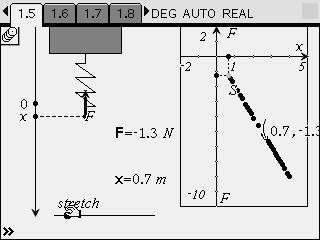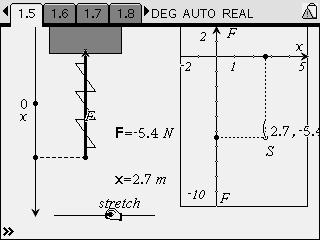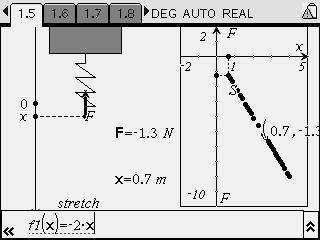# Activities

•##### Download

•• ##### Subject Area

• Science: Physics: Kinematics

• ##### Author9-12

15 Minutes

• ##### Device
• TI-Nspire™
• TI-Nspire™ CAS
• ##### Software

TI-Nspire™
TI-Nspire™ CAS

1.7

• ##### Report an Issue

Bell Ringer: Determining the Angle of a Ramp#### Activity Overview

Students use precompiled data to calculate the angle of a ramp from the displacement of a ball rolling on the ramp.

#### Key Steps

•Students use a precompiled data set to determine the angle of a ramp. The data set contains position vs. time data for a ball rolling up and down a ramp.

•Students fit a parabola to the position vs. time graph. They use the characteristics of the data set (i.e., the vertex of the data points) to predict the equation that best fits the data.

•Finally, students use the best-fit equation and the kinematic equations to calculate the angle of the ramp.